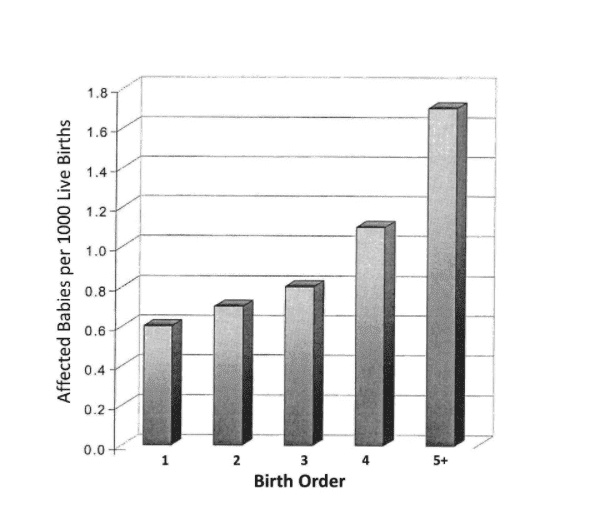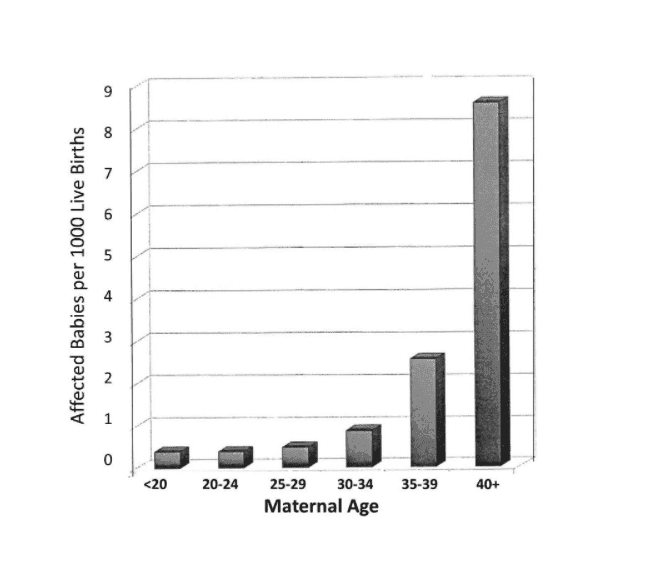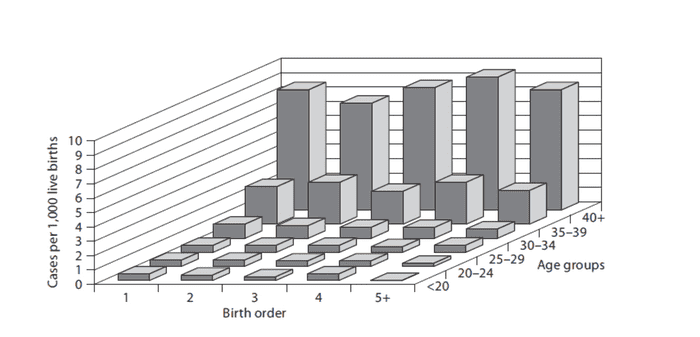# Multivariate Regression vs Stratification for confounding

So say we want to see if child birth order causes down syndrome. Say we get that child birth order is statisitcally associated down syndrome.We know that this association could be explained by a third variable: age. Women that give birth to say their third child, is of course going to be older than when they gave birth to their first child. So Age is associated with birth order.

Age of mother alone when child is born is associated with down syndrome.So we suspect that age confounds the relationship between birth order and down syndrome.

A way to see if this is true is to fix the levels of the confounding variable, Age, and then produce groups within which the confounder does not vary. So within each stratum, the confounder Age cannot confound because it doesn't vary across the exposure outcome.This is the statification strategy and it makes perfect sense. For the fixed Age groups, we see that birth order has no effect at each stratum of age.

Now how would this work for regression? Say I have y~birthorder. This would give statistical significance.

Then I adjust for age. y~birthorder+age. Here birthorder should not be significant. The interpretation is that birth order is not significant when age is constant.

So this seems like its the exact same thing as stratification right? In stratification, the strata is held constant. In regression, interpeting the output for the coefficient of birthorder, we hold age constant as well.

What if age is a continuous variable? Then would it work? Or would I have to split it into 5 strata just like in the stratification example?

FactChecker
Gold Member
If you apply stepwise multiple regression, it should give you the result that you see in the plots. I would try a stepwise linear regression with the independent variables of log(age) and birth_order. It appears that the incidence of down syndrome grows exponentially with age, so taking the logarithm of age should make a reasonably linear relationship. Stepwise regression would first identify log(age) as the most significant variable and put it into the model. Then it will adjust both the down numbers and the birth_order numbers to remove the influence of log(age) from both of them. Then it would look for any statistically significant remaining residual variation that can be explained by the adjusted birth_order. It will give you the statistics of birth_order. My guess is that there will not be any remaining significance and will not put birth_order into the model.
It should give you the statistical results to support your theory.

•FallenApple
Dale
Mentor
2020 Award
So this seems like its the exact same thing as stratification right?
There is a subtle difference. With stratification you wind up with several categories and test whether there is some difference between categories. With regression you are specifically testing if that difference is linear. So there are fewer degrees of freedom for a regression than for stratification (with more than 2 strata)

•FallenApple
There is a subtle difference. With stratification you wind up with several categories and test whether there is some difference between categories. With regression you are specifically testing if that difference is linear. So there are fewer degrees of freedom for a regression than for stratification (with more than 2 strata)

So if one does not get a linear difference, that could just imply that there might be a difference, but that difference is not linear, or that there is no difference at all, we do not know.

On the other hand, if stratification shows a difference, then there is a difference and the problem is solved, no need to run the linear test.

FactChecker
Gold Member
So if one does not get a linear difference, that could just imply that there might be a difference, but that difference is not linear, or that there is no difference at all, we do not know.

On the other hand, if stratification shows a difference, then there is a difference and the problem is solved, no need to run the linear test.
Analysis of variance (ANOVA) should give you answers regarding which independent variables are the main source of variance and which are negligible. But it will not take full advantage of the trends you can clearly see, where there are several categories of increasing age and several categories of increasing birth order. To take full advantage of the trends, you should try to use linear regression. Take the logarithm of age to make the trend more linear. Even if it is not fully linear with the logarithm of age, I am confident that there will be a strong trend that the linear regression will give a statistically significant first order fit to.

Analysis of variance (ANOVA) should give you answers regarding which independent variables are the main source of variance and which are negligible. But it will not take full advantage of the trends you can clearly see, where there are several categories of increasing age and several categories of increasing birth order. To take full advantage of the trends, you should try to use linear regression. Take the logarithm of age to make the trend more linear. Even if it is not fully linear with the logarithm of age, I am confident that there will be a strong trend that the linear regression will give a statistically significant first order fit to.
Ah ok. So in ANOVA, I have to categorize age. ANOVA is a special case of linear regression but with continuous response and categorical independent variables.

If I do linear regression, then age doesn't have to be categorized. So its more accurate that way? Since it takes into account an infinite amount to categories due to age being continuous?

Also, so do we take logarithms when the plot is upward curving? Say we don't know its exponential to be sure, but something slower but faster than linear.

FactChecker
Gold Member
Ah ok. So in ANOVA, I have to categorize age. ANOVA is a special case of linear regression but with continuous response and categorical independent variables.

If I do linear regression, then age doesn't have to be categorized. So its more accurate that way?

Also, so do we take logarithms when the plot is upward curving? Say we don't know its exponential to be sure, but something slower but faster than linear.
ANOVA is not a special case of regression analysis. General ANOVA does not depend on or take advantage of the age trend of your categories. It treats them like categories that can not be put in increasing factor order. In your data, the trend with increasing age is very obvious but general ANOVA would not take full advantage of that. There are some ANOVA(?) techniques that are designed to apply to data with factor levels that are ordered (see https://link.springer.com/article/10.1007/s13253-014-0170-5 ) . I don't have any experience with them.
Yes, regression should be more powerful for your case. You would not have to put the data into categories. The upward trend with age looks exponential to me and the logarithm should bring it closer to linear. It doesn't have to be linear to use linear regression; it's just that the fit to data will not be as good if it is not linear. But the trend is so obvious that linear regression should work very well.

•FallenApple
Dale
Mentor
2020 Award
So if one does not get a linear difference, that could just imply that there might be a difference, but that difference is not linear, or that there is no difference at all, we do not know.
That is correct. That is one reason to check your residuals. If there is a trend in the residuals then it would indicate that there might be a nonlinear difference.

On the other hand, if stratification shows a difference, then there is a difference and the problem is solved, no need to run the linear test.
You could take that approach, but I personally dislike it. I have a strong preference for more parsimonious models, so to me the fact that the stratified approach has so many more degrees of freedom is a big negative for that approach.

Also, stratification itself is a sketchy process. It is usually hard to justify the strata chosen and the choice often affects the results.

I would tend to start with a linear fit, and add terms if the residuals show a trend.

•FallenApple and FactChecker
ANOVA is not a special case of regression analysis. General ANOVA does not depend on or take advantage of the age trend of your categories. It treats them like categories that can not be put in increasing factor order. In your data, the trend with increasing age is very obvious but general ANOVA would not take full advantage of that. There are some ANOVA(?) techniques that are designed to apply to data with factor levels that are ordered (see https://link.springer.com/article/10.1007/s13253-014-0170-5 ) . I don't have any experience with them.
Yes, regression should be more powerful for your case.

I think you are talking about the sequential F tests. The one that uses type1 sum of squares. I'm not sure if it would give me the results though. Because type1SS uses the variability in the response without accounting for the possible confounder if the predictor of interest is entered first.

That is correct. That is one reason to check your residuals. If there is a trend in the residuals then it would indicate that there might be a nonlinear difference.

You could take that approach, but I personally dislike it. I have a strong preference for more parsimonious models, so to me the fact that the stratified approach has so many more degrees of freedom is a big negative for that approach.

Also, stratification itself is a sketchy process. It is usually hard to justify the strata chosen and the choice often affects the results.

I would tend to start with a linear fit, and add terms if the residuals show a trend.

Got it.

What would I do if I end up with collinearity though? So say x1 and x2 are highly correlated. I have a situation where the regression output for y~x1+x2 would give insignificant p values for both of them. But for the Ftest, using Anova(model) in R, I get that x1 is significant but x2 is not. This makes sense in that it is a type1 sum of squares. So SS(x1) used to calculate the p value the first term in the anova table. Then SS(x2|x1) is used to caculate the second line. But the problem with this is that I was supposed to be controlling for the confounding in the first place. SS(x1) doesn't account for confounding.

Then would linear regression still be valid? Can I just say that x1 is not significant after accounting for x2, using the wald test for linear regression?

FactChecker
Gold Member
I think you are talking about the sequential F tests. The one that uses type1 sum of squares. I'm not sure if it would give me the results though. Because type1SS uses the variability in the response without accounting for the possible confounder if the predictor of interest is entered first.
Not in that post. Sequential F tests are a standard part of the stepwise multiple regression, but not really relevant to the issue of using factors of increasing levels in an ANOVA.

IMHO you are overthinking this. Instead of all this guessing, you can probably try a sequential regression in about an hour and see what happens and if you are satisfied with the results.

Not in that post. Sequential F tests are a standard part of the stepwise multiple regression, but not really relevant to the issue of using factors of increasing levels in an ANOVA.

IMHO you are overthinking this. Instead of all this guessing, you can probably try a sequential regression in about an hour and see what happens and if you are satisfied with the results.

It was an example I found on this website:
http://sphweb.bumc.bu.edu/otlt/mph-...ding-em/bs704-ep713_confounding-em_print.html

I actually don't have the data set to analyze. But I often find helpful to discuss these concepts in general so in a different situation, but similarly suited data, I can apply the methods.

FactChecker
Gold Member
It was an example I found on this website:
http://sphweb.bumc.bu.edu/otlt/mph-...ding-em/bs704-ep713_confounding-em_print.html

I actually don't have the data set to analyze. But I often find helpful to discuss these concepts in general so in a different situation, but similarly suited data, I can apply the methods.
Oh. I understand now. You have been using a hypothetical example from an article. That example is made extreme to illustrate a point and to be obvious to the reader.
•